## Example Questions

← Previous 1 3 4 5 6

### Example Question #1 : How To Find The Length Of A Radius

In a large field, a circle with an area of 144π square meters is drawn out. Starting at the center of the circle, a groundskeeper mows in a straight line to the circle's edge. He then turns and mows ¼ of the way around the circle before turning again and mowing another straight line back to the center. What is the length, in meters, of the path the groundskeeper mowed?

24 + 6π

24 + 36π

24π

12 + 36π

12 + 6π

24 + 6π

Explanation:

Circles have an area of πr2, where r is the radius. If this circle has an area of 144π, then you can solve for the radius:

πr2 = 144π

r 2 = 144

r =12

When the groundskeeper goes from the center of the circle to the edge, he's creating a radius, which is 12 meters.

When he travels ¼ of the way around the circle, he's traveling ¼ of the circle's circumference. A circumference is 2πr. For this circle, that's 24π meters. One-fourth of that is 6π meters.

Finally, when he goes back to the center, he's creating another radius, which is 12 meters.

In all, that's 12 meters + 6π meters + 12 meters, for a total of 24 + 6π meters.

### Example Question #86 : Circles

Two concentric circles have circumferences of 4π and 10π.  What is the difference of the radii of the two circles?

3

5

6

7

4

3

Explanation:

The circumference of any circle is 2πr, where r is the radius.

Therefore:

The radius of the smaller circle with a circumference of 4π is 2 (from 2πr = 4π).

The radius of the larger circle with a circumference of 10π is 5 (from 2πr = 10π).

The difference of the two radii is 5-2 = 3.

### Example Question #87 : CirclesIn the figure above, rectangle ABCD has a perimeter of 40. If the shaded region is a semicircle with an area of 18π, then what is the area of the unshaded region?

336 – 36π

96 – 18π

336 – 18π

96 – 36π

204 – 18π

96 – 18π

Explanation:

In order to find the area of the unshaded region, we will need to find the area of the rectangle and then subtract the area of the semicircle. However, to find the area of the rectangle, we will need to find both its length and its width. We can use the circle to find the length of the rectangle, because the length of the rectangle is equal to the diameter of the circle.

First, we can use the formula for the area of a circle in order to find the circle's radius. When we double the radius, we will have the diameter of the circle and, thus, the length of the rectangle. Then, once we have the rectangle's length, we can find its width because we know the rectangle's perimeter.

Area of a circle = πr2

Area of a semicircle = (1/2)πr2 = 18π

Divide both sides by π, then multiply both sides by 2.

r2 = 36

Take the square root.

r = 6.

The radius of the circle is 6, and therefore the diameter is 12. Keep in mind that the diameter of the circle is also equal to the length of the rectangle.

If we call the length of the rectangle l, and we call the width w, we can write the formula for the perimeter as 2l + 2w.

perimeter of rectangle = 2l + 2w

40 = 2(12) + 2w

Subtract 24 from both sides.

16 = 2w

w = 8.

Since the length of the rectangle is 12 and the width is 8, we can now find the area of the rectangle.

Area = l x w = 12(8) = 96.

Finally, to find the area of just the unshaded region, we must subtract the area of the circle, which is 18π, from the area of the rectangle.

area of unshaded region = 96 – 18π.

The answer is 96 – 18π.

### Example Question #88 : Circles

Consider a circle centered at the origin with a circumference of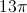. What is the x value when y = 3? Round your answer to the hundreths place.

5.778

10.00

5.8

5.77

5.77

Explanation:

The formula for circumference of a circle is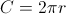, so we can solve for r: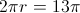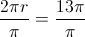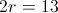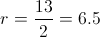We now know that the hypotenuse of the right triangle's length is 13.5. We can form a right triangle from the unit circle that fits the Pythagorean theorem as such: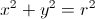Or, in this case: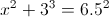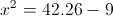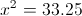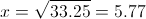### Example Question #1 : How To Find The Length Of A Radius

A circle has an area of 36π inches. What is the radius of the circle, in inches?

18

36

9

6

6

Explanation:

We know that the formula for the area of a circle is πr2. Therefore, we must set 36π equal to this formula to solve for the radius of the circle.

36π = πr2

36 = r2

6 = r

### Example Question #3 : How To Find The Length Of A Radius

Circle X is divided into 3 sections: A, B, and C. The 3 sections are equal in area. If the area of section C is 12π, what is the radius of the circle?Circle X

6

7

√12

4

6

Explanation:

Find the total area of the circle, then use the area formula to find the radius.

Area of section A = section B = section C

Area of circle X = A + B + C = 12π+ 12π + 12π = 36π

Area of circle =  where r is the radius of the circle

36π = πr2

36 = r2

√36 = r

6 = r

### Example Question #4 : How To Find The Length Of A Radius

The specifications of an official NBA basketball are that it must be 29.5 inches in circumference and weigh 22 ounces.  What is the approximate radius of the basketball?

9.39 inches

4.70 inches

3.06 inches

14.75 inches

5.43 inches

4.70 inches

Explanation:

To Find your answer, we would use the formula:  C=2πr. We are given that C = 29.5. Thus we can plug in to get  [29.5]=2πr and then multiply 2π to get 29.5=(6.28)r.  Lastly, we divide both sides by 6.28 to get 4.70=r.   (The information given of 22 ounces is useless)

### Example Question #8 : How To Find The Length Of A Radius

A circle with center (8, 5) is tangent to the y-axis in the standard (x,y) coordinate plane. What is the radius of this circle?

5

8

16

4

8

Explanation:

For the circle to be tangent to the y-axis, it must have its outer edge on the axis. The center is 8 units from the edge.

### Example Question #10 : How To Find The Length Of A Radius

A circle has an area of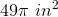. What is the radius of the circle, in inches?

49 inches

16 inches

24.5 inches

7 inches

14 inches

7 inches

Explanation:

We know that the formula for the area of a circle is πr2. Therefore, we must set 49π equal to this formula to solve for the radius of the circle.

49π = πr2

49 = r2

7 = r

### Example Question #1 : Radius

A certain circle has a circumference (in units) that is two times the area (in units squared). What is the radius of the circle?Explanation:

The formula for the area of a circle is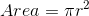The formula for the circumference of a circle is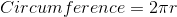Because the circumference is twice the area, your new formula is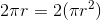You can divide both sides by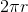, giving you the answer: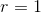← Previous 1 3 4 5 6